# 1998 seminars

## 08/01/2021, Friday, 17:00–18:00 Europe/Lisbon — Online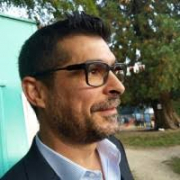Pedro Vaz, Université Catholique de Louvain, Belgium
Categorification of Verma Modules in low-dimensional topology

In this talk I will review the program of categorification of Verma modules and explain their applications to low-dimensional topology, namely to the construction of Khovanov invariants for links in the solid torus via a categorification of the blob algebra.

The material presented spreads along several collaborations with Abel Lacabanne, and Grégoire Naisse.

### Video

pedro-vaz-slides.pdf

## 15/01/2021, Friday, 17:00–18:00 Europe/Lisbon — OnlineBrent Pym, McGill University
Multiple zeta values in deformation quantization

In 1997, Kontsevich gave a universal solution to the deformation quantization problem in mathematical physics: starting from any Poisson manifold (the classical phase space), it produces a noncommutative algebra of quantum observables by deforming the ordinary multiplication of functions. His formula is a Feynman expansion whose Feynman integrals give periods of the moduli space of marked holomorphic disks. I will describe joint work with Peter Banks and Erik Panzer, in which we prove that Kontsevich's integrals evaluate to integer-linear combinations of multiple zeta values, building on Francis Brown's theory of polylogarithms on the moduli space of genus zero curves.

Pym slides.pdf

## 22/01/2021, Friday, 17:00–18:00 Europe/Lisbon — Online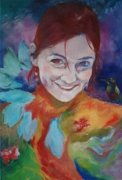Renee Hoekzema, University of Oxford
Manifolds with odd Euler characteristic and higher orientability

Orientable manifolds have even Euler characteristic unless the dimension is a multiple of 4. I give a generalisation of this theorem: $k$-orientable manifolds have even Euler characteristic (and in fact vanishing top Wu class), unless their dimension is $2^{k+1}m$ for some integer $m$. Here we call a manifold $k$-orientable if the $i^{\rm{th}}$ Stiefel-Whitney class vanishes for all 0 < $i$ < $2^k$. This theorem is strict for $k=0,1,2,3$, but whether there exist 4-orientable manifolds with an odd Euler characteristic is a new open question. Such manifolds would have dimensions that are a multiple of 32. I discuss manifolds of dimension high powers of 2 and present the results of calculations on the cohomology of the second Rosenfeld plane, a special 64-dimensional manifold with odd Euler characteristic.

### Video

renee-hoekzema-slides.pdf

## 29/01/2021, Friday, 17:00–18:00 Europe/Lisbon — OnlineGerbes are geometric objects describing the third integer cohomology group of a manifold and the B-field in string theory; they can essentially be understood as bundles of categories whose fibre is equivalent to the category of vector spaces. Starting from a hands-on example, I will explain gerbes and their categorical features. The main topic of this talk will then be the study of symmetries of gerbes in a universal manner. We will see that these symmetries are completely encoded in an extension of smooth 2-groups. In the last part, I will survey how this construction can be used to provide a new smooth model for the string group, via a theory of group extensions in $\infty$-topoi.

Bunk-slides.pdf

## 12/02/2021, Friday, 17:00–18:00 Europe/Lisbon — Online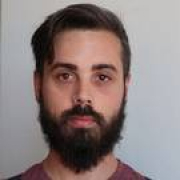Florio M. Ciaglia, Max Planck Institute for Mathematics in the Sciences, Leipzig
A groupoid-based perspective on quantum mechanics

In this talk, I will expound a point of view on the theoretical investigation of the foundations and mathematical formalism of quantum mechanics which is based on Schwinger’s “Symbolism of atomic measurement”  on the physical side, and on the notion of groupoid on the mathematical side. I will start by reviewing the “development” of quantum mechanics and its formalism starting from Schrödinger’s wave mechanics, passing through the Hilbert space quantum mechanics, and arriving at the $C^∗$-algebraic formulation of quantum mechanics in order to give an intuitive idea of what is the “place” of the groupoid-based approach to quantum theories presented here. Then, after (what I hope will be) a highly digestible introduction to the notion of groupoid, I will review two historic experimental instances in which the shadow of the structure of groupoid may be glimpsed, namely, the Ritz-Rydberg combination principle, and the Stern-Gerlach experiment. The last part of the talk will be devoted to building a bridge between the groupoid-based approach to quantum mechanics and the more familiar $C^∗$-algebraic one by analysing how to obtain a (possibly) non-commutative algebra out of a given groupoid. Two relevant examples will be discussed, and some comment on future directions (e.g., the composition of systems) will close the talk. The material presented is part of an ongoing project developed together with Dr. F. Di Cosmo, Prof. A. Ibort, and Prof. G. Marmo. In particular, the discrete-countable theory has already appeared in [1, 2, 3, 4, 5, 6, 7].

References

 F. M. Ciaglia, F. Di Cosmo, A. Ibort, and G. Marmo. Evolution of Classical and Quantum States in the Groupoid Picture of Quantum Mechanics. Entropy, 11(22):1292 – 18, 2020.

 F. M. Ciaglia, F. Di Cosmo, A. Ibort, and G. Marmo. Schwinger’s Picture of Quantum Mechanics. International Journal of Geometric Methods in Modern Physics, 17(04):2050054 (14), 2020.

 F. M. Ciaglia, F. Di Cosmo, A. Ibort, and G. Marmo. Schwinger’s Picture of Quantum Mechanics IV: Composition and independence. International Journal of Geometric Methods in Modern Physics, 17(04):2050058 (34), 2020.

 F. M. Ciaglia, A. Ibort, and G. Marmo. A gentle introduction to Schwinger’s formulation of quantum mechanics: the groupoid picture. Modern Physics Letters A, 33(20):1850122–8, 2018.

 F. M. Ciaglia, A. Ibort, and G. Marmo. Schwinger’s Picture of Quantum Mechanics I: Groupoids. International Journal of Geometric Methods in Modern Physics, 16(08):1950119 (31), 2019.

 F. M. Ciaglia, A. Ibort, and G. Marmo. Schwinger’s Picture of Quantum Mechanics II: Algebras and Observables. International Journal of Geometric Methods in Modern Physics, 16(09):1950136 (32), 2019.

 F. M. Ciaglia, A. Ibort, and G. Marmo. Schwinger’s Picture of Quantum Mechanics III: The Statistical Interpretation. International Journal of Geometric Methods in Modern Physics, 16(11):1950165 (37), 2019.

 J. Schwinger. Quantum Mechanics, Symbolism of Atomic Measurements. Springer-Verlag, Berlin, 2001.

### Video

florio-ciaglia-slides.pdf

Florio-Ciaglia-annotated-slides

## 19/02/2021, Friday, 17:00–18:00 Europe/Lisbon — OnlineDavid Reutter, Max Planck Institute for Mathematics
Semisimple topological field theories in even dimensions

A major open problem in quantum topology is the construction of an oriented 4-dimensional topological quantum field theory (TQFT) in the sense of Atiyah-Segal which is sensitive to exotic smooth structure. More generally, how much manifold topology can a TQFT see?

In this talk, I will answer this question for semisimple field theories in even dimensions — I will sketch a proof that such field theories can at most see the stable diffeomorphism type of a manifold and conversely, that if two sufficiently finite manifolds are not stably diffeomorphic then they can be distinguished by semisimple field theories. In this context, 'semisimplicity' is a certain algebraic condition applying to all currently known examples of vector-space-valued TQFTs, including 'unitary field theories’, and 'once-extended field theories' which assign algebras or linear categories to codimension 2 manifolds. I will discuss implications in dimension 4, such as the fact that oriented semisimple field theories cannot see smooth structure, while unoriented ones can.

Throughout, I will use the Crane-Yetter field theory associated to a ribbon fusion category as a guiding example.

This is based on arXiv:2001.02288 and joint work in progress with Chris Schommer-Pries.

### Video

David-Reutter-slides.pdf

## 26/02/2021, Friday, 17:00–18:00 Europe/Lisbon — OnlineBen Elias, University of Oregon
Introduction to the Hecke category and the diagonalization of the full twist

The group algebra of the symmetric group has a large commutative subalgebra generated by Young-Jucys-Murphy elements, which acts diagonalizably on any irreducible representation. The goal of this talk is to give an accessible introduction to the categorification of this story. The main players are: Soergel bimodules, which categorify the Hecke algebra of the symmetric group; Rouquier complexes, which categorify the braid group where Young-Jucys-Murphy elements live; and the Elias-Hogancamp theory of categorical diagonalization, which allows one to construct projections to "eigencategories."

### Video

ben-elias-slides.pdf

## 05/03/2021, Friday, 14:00–15:00 Europe/Lisbon — Online

Owen Gwilliam, University of Massachusetts, Amherst
Bulk-boundary correspondences with factorization algebras

Factorization algebras provide a flexible language for describing the observables of a perturbative QFT, as shown in joint work with Kevin Costello. Those constructions extend to a manifold with boundary for a special class of theories. I will discuss work with Eugene Rabinovich and Brian Williams that includes, as an example, a perturbative version of the correspondence between chiral ${\rm U}(1)$ currents on a Riemann surface and abelian Chern-Simons theory on a bulk 3-manifold, but also includes a systematic higher dimensional version for higher abelian CS theory on an oriented smooth manifold of dimension $4n+3$ with boundary a complex manifold of complex dimension $2n+1$. Given time, I will discuss how this framework leads to a concrete construction of the center of higher enveloping algebras of Lie algebras, in work with Greg Ginot and Brian Williams.

### Video

Gwilliam_slides.pdf

Note the unusual time.

## 12/03/2021, Friday, 17:00–18:00 Europe/Lisbon — Online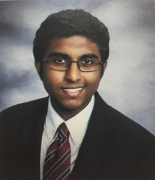Ikshu Neithalath, UCLA, California
Skein Lasagna modules of 2-handlebodies

Morrison, Walker and Wedrich recently defined a generalization of Khovanov-Rozansky homology to links in the boundary of a 4-manifold. We will discuss recent joint work with Ciprian Manolescu on computing the "skein lasagna module," a basic part of MWW's invariant, for a certain class of 4-manifolds.

ikshu_slides.pdf

## 19/03/2021, Friday, 17:00–17:40 Europe/Lisbon — OnlineFabio di Cosmo, Instituto de Ciencias Matemáticas, Madrid
Statistical Interpretation in the Schwinger’s picture of Quantum Mechanics

In this talk I will illustrate some ideas about the statistical interpretation in the Schwinger’s picture of Quantum Mechanics. After a brief introduction on the postulates assumed in this framework, I will recall the basic ingredients of Connes’ non commutative integration theory. This language allows me to define, on one hand quantum measures on the groupoid associated with the quantum systems, and on the other weights on the corresponding groupoid von-Neumann algebra. In particular, quantum measures are a generalization of measures on sigma-algebras which is suited for the description of interference phenomena. Then, the final part of the talk will be devoted to the statistical interpretation associated with both situations.

fabio-di-cosmo-slides.pdf

First part of a double session, followed by a 20 minute break for coffee and discussion, before the second speaker, Pedro Resende.

## 19/03/2021, Friday, 18:00–18:40 Europe/Lisbon — Online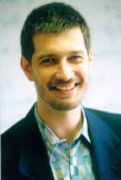Pedro Resende, Instituto Superior Técnico, Lisbon
An abstract theory of physical measurements

Since its early days, quantum mechanics has forced physicists to consider the interaction between quantum systems and classically described experimental devices — a fundamental tenet for Bohr was that the results of measurements need to be communicated using the language of classical physics.

Several decades of progress have led to improved understanding, but the tension between “quantum” and “classical” persists. Ultimately, how is classical information extracted from a measurement? Is classical information fundamental, as in Wheeler’s “it from bit”? In this talk, which is based on ongoing work , I approach the problem mathematically by considering spaces whose points are measurements, abstractly conceived in terms of the classical information they produce. Concretely, measurement spaces are stably Gelfand quantales  equipped with a compatible sober topology, but essentially their definition hinges on just two binary operations, called composition and disjunction, whose intuitive meanings are fairly clear. Despite their simplicity, these spaces have interesting mathematical properties. C*-algebras yield measurement spaces of “quantum type,” and Lie groupoids give us spaces of “classical type,” such as those which are associated with a specific experimental apparatus. The latter also yield a connection to Schwinger’s selective measurements, which have been recast in groupoid language by Ciaglia et al.

An interaction between the two types, providing a mathematical approach to Bohr’s quantum/classical split, can be described in terms of groupoid (or Fell bundle) C*-algebras as in . I will illustrate the basic ideas with simple examples, such as spin measurements performed with a Stern–Gerlach apparatus.

### References

1. P. Resende, An abstract theory of physical measurements (2021).
2. P. Resende, The many groupoids of a stably Gelfand quantale, J. Algebra 498 (2018), 197–210.
3. P. Resende, Quantales and Fell bundles, Adv. Math. 325 (2018), 312–374, MR3742593.slidesv6post.pdf

Second part of a double session, followed by a 20 minute discussion period.

## 09/04/2021, Friday, 17:00–18:00 Europe/Lisbon — Online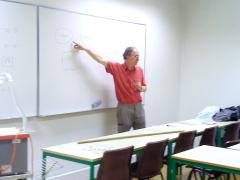Roger Picken, Instituto Superior Técnico and CAMGSD
Link invariants from finite crossed modules and a lifting of the Eisermann invariant

This talk is based on work with João Faria Martins (Univ. Leeds)  and several projects with students. I will describe the construction of an invariant of tangles and framed tangles which takes values in an arbitrary crossed module of finite groups. This involves the fundamental crossed module associated to a natural topological pair coming from a knot diagram, and a suitable class of morphisms from this fundamental crossed module to the chosen finite crossed module. Our construction includes all rack and quandle cohomology (framed) link invariants, as well as the Eisermann invariant of knots [2-3], for which we also find a lifting. The Eisermann invariant detects information about a suitable choice of meridian and longitude in the knot complement boundary.

 João Faria Martins and Roger Picken: Link invariants from finite categorical groups, Homology, Homotopy and Applications, 17(2) (2015), 205–233; arXiv:1301.3803v2 [math.GT], arXiv:1612.03501v1 [math.GT]
 M. Eisermann: Knot colouring polynomials, Pacific J. Math. 231 (2007), no. 2, 305–336.
 M. Eisermann: Homological characterization of the unknot, J. Pure Appl. Algebra 177 (2003), no. 2, 131–157.

### Video

roger-picken-slides.pdf

## 16/04/2021, Friday, 17:00–18:00 Europe/Lisbon — OnlineGiordano Cotti, Grupo de Física Matemática, Universidade de Lisboa
Quantum differential equations, qKZ difference equations, and helices.

Quantum differential equations (qDEs) are a rich object attached to complex smooth projective varieties. They encode information on their enumerative geometry, topology and (conjecturally) on their algebraic geometry. In occasion of the 1998 ICM in Berlin, B.Dubrovin conjectured an intriguing connection between the enumerative geometry of a Fano manifold $X$ with algebro-geometric properties of exceptional collections in the derived category $D_b(X)$. Under the assumption of semisimplicity of the quantum cohomology of $X$, the conjecture prescribes an explicit form for local invariants of $QH^*(X)$, the so-called “monodromy data”, in terms of Gram matrices and characteristic classes of objects of exceptional collections. In this talk I will discuss an equivariant analog of these relations, focusing on the example of projective spaces. The study of the equivariant quantum differential equations for partial flag varieties has been initiated by V.Tarasov and A.Varchenko in 2017. They discovered the existence of a system of compatible qKZ difference equations, which have made the study of the quantum differential equations easier than in the non-equivariant case. I will establish relations between the monodromy data of the joint system of the equivariant qDE and qKZ equations for $\mathbb{P}^n$ and characteristic classes of objects of the derived category of T-equivariant coherent sheaves on $\mathbb{P}^n$.

Based on joint works with B.Dubrovin, D.Guzzetti and A.Varchenko.

## 07/05/2021, Friday, 17:00–18:00 Europe/Lisbon — OnlineChristian Saemann, Heriot-Watt University
Adjusted Higher Gauge Theory: Connections and Parallel Transport

Ordinary higher gauge theory suffers from the problem that all the curvature forms but the top degree one, which are called fake curvatures, have to vanish. If this condition was omitted, the gauge structure, the underlying higher principal bundle and the corresponding parallel transport would be inconsistent. For vanishing fake curvatures, however, one can locally gauge away the non-abelian parts of the higher connection, ending up with a connection on an abelian gerbe. This is clearly unsatisfactory for non-topological higher gauge theories. A solution to this problem is what we call "adjusted higher gauge theory", in which the usual definition of the curvatures is adjusted by additional data. This lifts the requirement for vanishing fake curvatures. Moreover, it matches constructions of theoretical physicists in the context of supergravity. In this talk, I will review the above points and say a few words about my motivation for studying adjusted higher gauge theories.

### Video

christian-saemann-slides.pdf

## 14/05/2021, Friday, 09:00–10:00 Europe/Lisbon — OnlineSi Li, Tsinghua University
Geometry of Localized Effective Theories and Algebraic Index

We describe a general framework to study the quantum geometry of $\sigma$-models when they are effectively localized to small quantum fluctuations around constant maps. Such effective theories have exact descriptions at all loops in terms of target geometry and can be rigorously formulated. We illustrate this idea by the example of topological quantum mechanics which will lead to an explicit construction of the universal trace map on periodic cyclic chains of matrix Weyl algebras. As an application, we explain how to implement the idea of exact semi-classical approximation into a proof of the algebraic index theorem using Gauss Manin connection.

This is joint work with Zhengping Gui and Kai Xu.

Zhengping Gui, Si Li, Kai Xu,
Geometry of Localized Effective Theories, Exact Semi-classical Approximation and the Algebraic Index
https://arxiv.org/pdf/1911.11173

Li Si slides.pdf

## 21/05/2021, Friday, 17:00–18:00 Europe/Lisbon — OnlineBruce Bartlett, Stellenbosch University
Asymptotics of the classical and quantum $6j$ symbols

The classical (resp. quantum) $6j$ symbols are real numbers which encode the associator information for the tensor category of representations of $SU(2)$ (resp. the quantum group of $SU(2)$ at level $k$). They form the building blocks for the Turaev-Viro $3$-dimensional TQFT. I will review the intriguing asymptotic formula for these symbols in terms of the geometry of a Euclidean tetrahedron (in the classical case) or a spherical tetrahedron (in the quantum case), due to Ponzano-Regge and Taylor-Woodward respectively. There is a wonderful integral formula for the square of the classical $6j$ symbols as a group integral over $SU(2)$, and I will report on investigations into a similar conjectural integral formula for the quantum $6j$ symbols. In the course of these investigations, we observed and proved a certain reciprocity formula for the Wigner derivative for spherical tetrahedra. Joint with Hosana Ranaivomanana.

### Video

Bruce Bartlett slides.pdf

## 28/05/2021, Friday, 17:00–18:00 Europe/Lisbon — OnlineDmitry Melnikov, International Institute of Physics, Natal
Entanglement and complexity from TQFT

In the 1990s Aravind proposed that topological links can be used to classify different patterns of quantum entanglement. One way this connection can be investigated is through an appropriate quantum mechanical definition of knots. I will start from the category theory definition of a TQFT and derive a relation between measures of quantum entanglement and topological invariants of links. We will see how patterns of quantum entanglement emerge in the TQFT picture. Meanwhile, complexity is a complementary measure of quantum correlations. In the TQFT case, it can also be related to topological invariants. I will discuss a few definitions of complexity for several families of knots and links.

### Video

dmitry-melnikov-slides.pdf

## 04/06/2021, Friday, 17:00–18:00 Europe/Lisbon — OnlineIngmar Saberi, Ludwig Maximilian University, Munich
Twists of supergravity theories via algebraic geometry

Twists of supersymmetric field theories have been the source of an enormous amount of new mathematics, including (just for example) Seiberg-Witten theory and mirror symmetry. It is reasonable to expect that twists of supergravity theories will exhibit even richer structure, but they remain comparatively unexplored, largely due to their intricacy. For example, a holomorphically twisted version of the AdS/CFT correspondence was proposed by Kevin Costello and Si Li, motivated by constructions in topological string theory and the work of Bershadsky-Cecotti-Ooguri-Vafa; Costello and Li conjectured a connection between their version of BCOV theory and the type IIB supergravity theory, but did not verify this connection directly. I will show that the pure spinor superfield technique, which has been known for some time in the physics literature, can be used to elegantly and economically construct supersymmetric theories, as well as to swiftly compute their twists. In each case, the resulting structures are governed by the classical algebraic geometry of certain affine varieties. If time permits, I'll discuss the examples of type IIB supergravity and eleven-dimensional supergravity in some detail.

### Video

Ingmar-Lisbon-notes.pdf

## 18/06/2021, Friday, 17:00–18:00 Europe/Lisbon — OnlineTheo Johnson-Freyd, Dalhousie University and Perimeter Institute
Higher S-matrices

Each fusion higher category has a "framed S-matrix" which encodes the commutator of operators of complementary dimension. I will explain how to construct and interpret this pairing, and I will emphasize that it may fail to exist if you drop semisimplicity requirements. I will then outline a proof that the framed S-matrix detects (non)degeneracy of the fusion higher category. This is joint work in progress with David Reutter.

### Video

Theo-Johnson-Freyd-slides

## 25/06/2021, Friday, 17:00–18:00 Europe/Lisbon — Online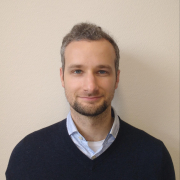Fabian Haiden, Mathematical Institute, University of Oxford
Categorical Kähler Geometry

This is a report on joint work in progress with L. Katzarkov, M. Kontsevich, and P. Pandit. The Homological Mirror Symmetry conjecture is stated as an equivalence of triangulated categories, one coming from algebraic geometry and the other from symplectic topology. An enhancement of the conjecture also identifies stability conditions (in the sense of Bridgeland) on these categories. We adopt the point of view that triangulated (DG/A-infinity) categories are non-commutative spaces of an algebraic nature. A stability condition can then be thought of as the analog of a Kähler class or polarization. Many, often still conjectural, constructions of stability conditions hint at a richer structure which we think of as analogous to a Kähler metric. For instance, a type of Donaldson and Uhlenbeck-Yau theorem is expected to hold. I will discuss these examples and common features among them, leading to a tentative definition.

### Video